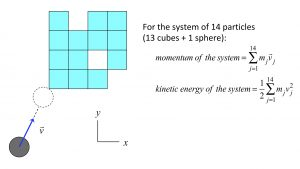# Momentum conservationA sphere moving on a smooth horizontal surface (the xy-plane) impacts a set of 13 cubes at rest on the same surface. This impacts leads to a number of subsequent impacts among these cubes. The resulting motion is quite complicated; however, since there are no forces acting on the system of the sphere + cubes in the x- and y-directions, we know that linear momentum for that system is conserved in both directions.

The animation below shows the results of a simulation of these impacts.As expected, both of the x- and y-components of linear momentum for the system are a constant, even through the times of impacts. It is easy to see that the individual momenta of the particles are NOT constant.

Another observation to make from these simulation results is that the energy in the system is NOT constant. A loss of energy occurs across each impact seen in the motion. Why is that?

In conclusion, conservation momentum does NOT imply conservation of energy (and, vice versa). This is a very important general concept to remember!